﻿ Wordcloud 实现云标签（2） - 易一网络科技|www.yeayee.com - Python3.x|34|3.4|Python基础|爬虫Crapy|Web建站Flask|Gui界面Tkinter|零基础学习

## 本站出品

预售广告位一处

微信号nemoon

### Wordcloud 实现云标签（2）

word_cloud 生成词云有两个方法。from text 和 from frequencies 。如果我们处理英文文档的话，就可以直接使用第一个方法，而不需要提前预处理文本，由于我们要做的是中文文本，所以我们必须自己分词，并处理为数组，使用第二种方法。

`#-*-coding:utf-8 -*-from os import pathfrom wordcloud import WordCloudimport matplotlib.pyplot as pltd = path.dirname(__file__)frequencies = [('易一',10),('网络',2),('科技',2),('测试',2),('样例',1)]wordcloud = WordCloud().fit_words(frequencies)plt.imshow(wordcloud)plt.axis("off")plt.show()# wordcloud = WordCloud(max_font_size=40, relative_scaling=.5).fit_words(frequencies)# plt.figure()# plt.imshow(wordcloud)# plt.axis("off")# plt.show()`
`运行代码得到结果（注释部分设定了字体大小和比例，可以不用） `然后对这张图进行处理。

```from os import pathfrom PIL import Imageimport numpy as npimport matplotlib.pyplot as pltfrom wordcloud import WordCloud, STOPWORDSd = path.dirname(__file__)# Read the whole text.text = open(path.join(d, 'alice.txt')).read()  # 获取当前运行目录的文本'alice.txt'# read the mask image# taken fromalice_mask = np.array(Image.open(path.join(d, "huge.jpg")))  # 原作者用胡歌的图片，那就用他吧wc = WordCloud(background_color="white", max_words=2000, mask=alice_mask,               stopwords=STOPWORDS.add("said"))# generate word cloud 生成wc.generate(text)# store to file 保存wc.to_file(path.join(d, "alice.png"))# showplt.imshow(wc)plt.axis("off")# plt.figure()plt.show()# plt.imshow(alice_mask, cmap=plt.cm.gray)  # 这句就是以灰度显示图片，也就是说任何图片都可以的# plt.axis("off")# plt.show()
```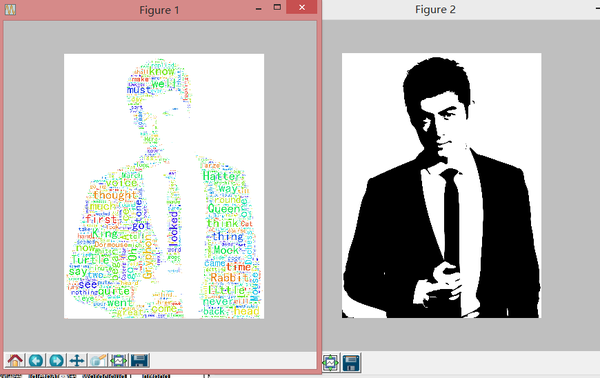`import numpy as npfrom PIL import Imagefrom os import pathimport matplotlib.pyplot as pltimport randomfrom wordcloud import WordCloud, STOPWORDSdef grey_color_func(word, font_size, position, orientation, random_state=None, **kwargs):    return "hsl(0, 0%%, %d%%)" % random.randint(60, 100)d = path.dirname(__file__)# read the mask image# taken frommask = np.array(Image.open(path.join(d, "huge.jpg")))# movie script of "a new hope"# May the lawyers deem this fair use.text = open("a_new_hope.txt").read()# preprocessing the text a little bittext = text.replace("HAN", "Han")text = text.replace("LUKE'S", "Luke")# adding movie script specific stopwordsstopwords = STOPWORDS.copy()stopwords.add("int")stopwords.add("ext")wc = WordCloud(max_words=1000, mask=mask, stopwords=stopwords, margin=10,               random_state=1).generate(text)# store default colored imagedefault_colors = wc.to_array()  # 默认色值plt.title("Custom colors")plt.imshow(wc.recolor(color_func=grey_color_func, random_state=3))wc.to_file("a_new_hope.png")plt.axis("off")# plt.figure()  # 默认的色值确实不行哈# plt.title("Default colors")# plt.imshow(default_colors)# plt.axis("off")plt.show()`
`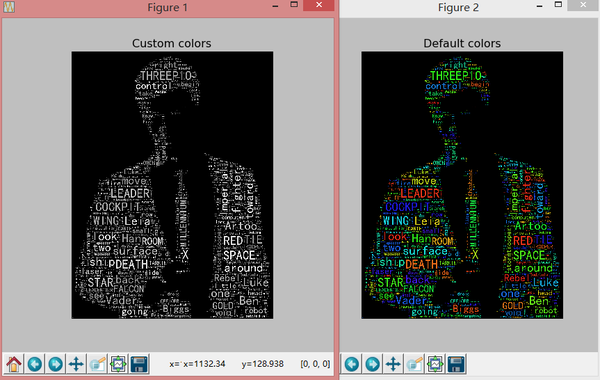`

```from os import pathfrom PIL import Imageimport numpy as npimport matplotlib.pyplot as pltfrom wordcloud import WordCloud, STOPWORDS, ImageColorGeneratord = path.dirname(__file__)# Read the whole text.text = open(path.join(d, 'alice.txt')).read()# read the mask / color image# taken from http://jirkavinse.deviantart.com/art/quot-Real-Life-quot-Alice-282261010alice_coloring = np.array(Image.open(path.join(d, "xhr.jpg")))wc = WordCloud(background_color="white", max_words=2000, mask=alice_coloring,               stopwords=STOPWORDS.add("said"),               max_font_size=40, random_state=42)# generate word cloudwc.generate(text)# create coloring from imageimage_colors = ImageColorGenerator(alice_coloring)# showplt.imshow(wc)plt.axis("off")plt.figure()# recolor wordcloud and show# we could also give color_func=image_colors directly in the constructorplt.imshow(wc.recolor(color_func=image_colors))plt.axis("off")plt.figure()plt.imshow(alice_coloring, cmap=plt.cm.gray)plt.axis("off")plt.show()
```
`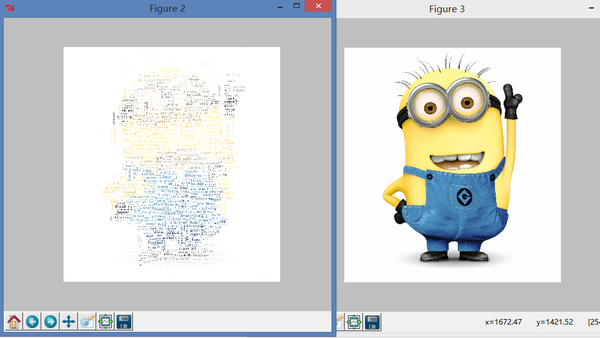效果还可以，但是和我想的…不太一样…我们尝试调整一些参数。……当然，最佳效果可能需要很多次的尝试才会出现。比如我将字号调大以后就会出现一些……奇怪的输出，难道是因为白色白的不够纯净吗。`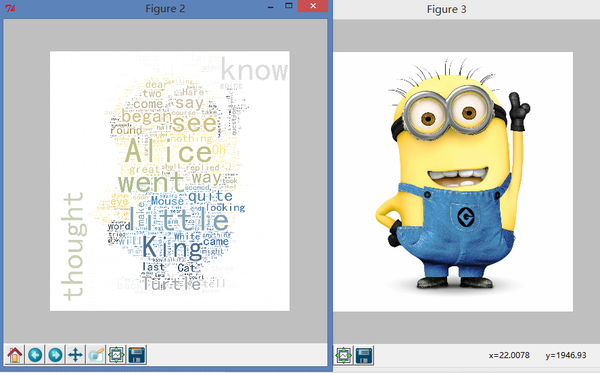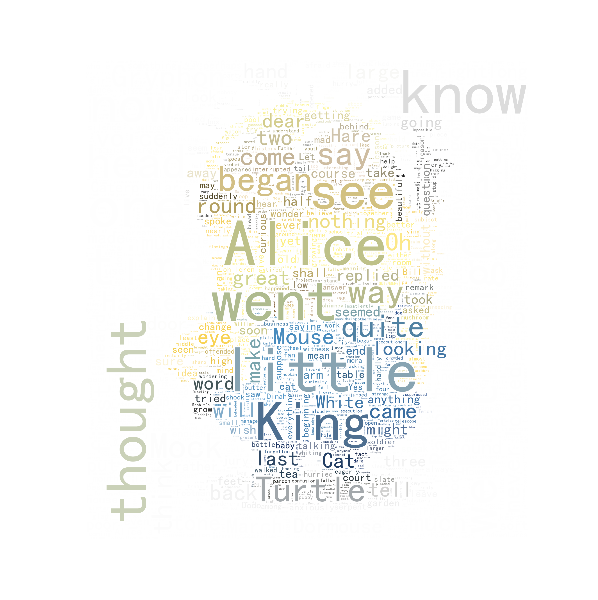总体上讲这样的配色已经比上面那种花花绿绿的配色好多了…至于为什么白色区域还会有词语，我刚看了一下小黄人的原图，白色区域的确不完全是 255,255,255 ，应该是这个原因。

``wc = WordCloud(background_color="white", max_words=2000, mask=alice_coloring,                stopwords=STOPWORDS.add("said"),                max_font_size=40, random_state=42,scale =1.5 ) ``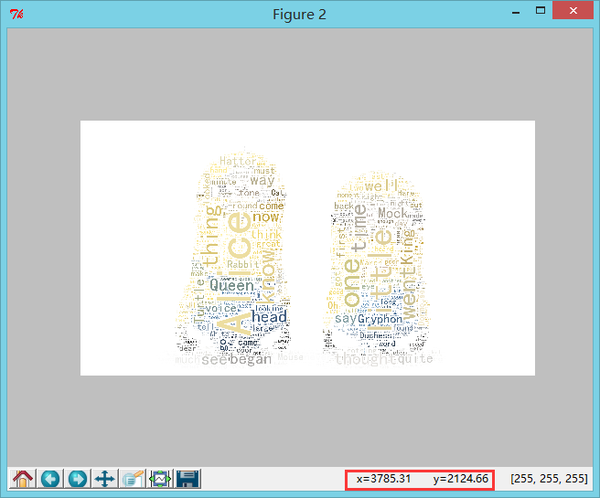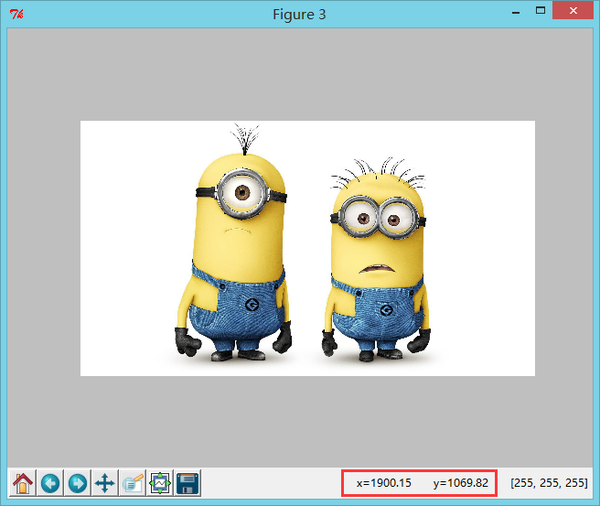可以看到输出的图片的确是原图的 2 倍。

1、FONT_PATH

2、中文要实现分词，输出为数组，使用 frequencies 方法

3、scale 的用法，由于程序运行时间较长，如果生成大幅图片会很慢，可以使用 scale 调节大小，但是会牺牲词语对形状的拟合度

pytagcloud ：

word_cloud：

#这一篇主要是对官方样例的重复，比较简单，代码也是现成的。图片可以根据喜好自己去找，就不提供这次用到的文件了。

著作权归作者所有。